# Polyominoes

Polyominoes are shapes formed from joining squares edge-to-edge, (like Tetris blocks) and this is my book about them:

When I started this project, the idea was to write one or two essays about what I could find out about polyominoes; it quickly grew into a more ambitious project. Right now, I hope to build a useful reference that looks at what is known about these simple shapes in a coherent way, without delving into higher math. This is a work in progress, so I expect these goals may shift.

As it stands, the book covers a substantial amount of material. Instead of giving a high-level summary, I will give some interesting findings. If this piques your interest; download the PDF above.

A domino is a polyomino with two cells. Regions whose sides all have even lengths can be tiled by dominoes. For regions without holes, it is not very remarkable (try to prove it!). But it is also true for regions with holes (of course, the holes need to have even sides).

It is not important where we put the holes. In fact, we can move the holes all the way up against the border and it still works. Of course, such a “hole”is not really a hole any more. This maneuver allows us to apply the theorem to figures which does not have all sides of even length.

Every region that can be tiled with dominoes either has at least two peaks, or a hole, or every tiling thereof has a flippable pair. (See the image below for examples.)A region with two peaks.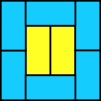A tiling with a flippable pair.A region with a hole.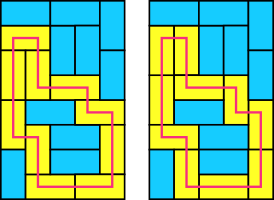Two tilings of a rectangle, with a cycle marked in yellow. The cycle is tiled differently in each rectangle. There are also other cycles, some of which overlap the one shown.

If a region has a unique tiling, it must have two peaks, and it cannot have any flippable pairs. In fact, it can have no cycles of dominoes, since cycles always have two tilings.

In a domino tiling of a region, we can predict locations of flippable pairs from the corners of that region. This means we can also predict the location of flippable pairs knowing where corners inside the figures form. The prediction is that a flippable pair will lie on a diagonal extended from the corner.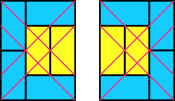The two tilings of a $3\times 4$ rectangle with one flippable pair. Diagonal lines drawn from the corners determine where the flippable pair should be.

There are at most two tilings of any rectangle by dominoes with exactly one flippable pair. The flippable pair occurs at or close to the center of the rectangle, where the diagonals lines drawn from the corners overlap. If there are two tilings, the one is a rotation of the other.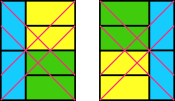Two tilings of a rectangle with exactly two non-overlapping flippable pairs.

There are also at most two tilings of any rectangle by dominoes with exactly two (non-overlapping) flippable pairs. These can be obtained from the tilings with one flippable pair by flipping that flippable pair.

Suppose we color a polyomino with a checkerboard coloring (that is, alternate cells in different colors), and that there is exactly one blue cell that meet each corner. (At corner cells, either one or three cells can meet, so either it is one blue cell, or one blue cell and two yellow cells.) Such a polyomino is tileable by dominoes.

Suppose we color the each cell in the plane with a color so that no matter how we place a polyomino, each cell has a different color. Such a coloring is called sufficient coloring for the polyomino. If such a coloring uses the minimum number of colors, the coloring is an optimal coloring for the polyomino, and the number of colors is called the chromatic number of the polyomino.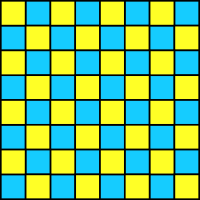The checkerboard coloring. Cells are alternatively colored with two different colors.

The checkerbaord coloring is an optimal coloring for the domino, so the chromatic number for the domino is 2.

The chromatic number for a $m \times n$ rectangle is $mn$ if and only if $m$ divides $n$ or vice versa. For example, we need 8 colors for a $2\times 3$ rectangle; we also need 8 colors for a $2\times 4$ rectangle. There are some results for other rectangles, but the general case seems open.VZ

There are polyominoes with $2n + 1$ cells whose chromatic numbers are $m^2$. For example, the V- and Z-pentominoes each require 9 colors. To see this, note that any two cells of a $3 \times 3$ square can be covered by either polyomino, so in any coloring these cells at least must be different. The coloring below shows 9 colors are enough.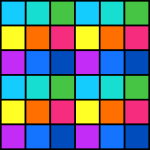A coloring using 9 colors.

The chromatic number is always bigger than or equal to the number of cells of the polyomino. If the chromatic number is equal, the polyomino is called efficient. There are 2 efficient polyominoes with 5 cells, 2 with 6 cells, and 1 with 7 cells. But there are many (dozens) with 8 and 9 cells. I don’t know whether efficient polyominoes with 10 cells are common or rare. My guess is rare, since the symmetries in colorings that account for the abundance of efficient polyominoes with 8 or 9 cells do not apply to colorings with 10 colors.

If we have a rectangles whose horizontal and vertical sides don’t share a common factor, that rectangle can tile any rectangle if it is big enough. Finding out what is “big enough” can be a very difficult problem.

We can never fit more than $\lfloor m/p \rfloor\lfloor n/p \rfloor$ squares with side $p$ in a $m \times n$ rectangle.

Let’s take an example to see how this works: How many $2\times 2$ squares can we fit into a $9 \times 9$? There are 81 cells in the big square, so it would be nice if we could fit 20. However, we cannot. Color the cells of the big square as shown. Now, no matter how we place a small square, it must always cover exactly one blue square. But there are only 16; therefor, it is impossible to fit more than 16 small squares in the bigger.

It is not known (in general) what is the maximum number of one type of rectangle that can fit into another. (To get a feel for why this could be difficult, consider that four $2 \times 3$ rectangles can fit into a $5 \times 5$ square. This arrangement is sometimes called a pinwheel pattern)

All L-shaped polyominoes with width 2 can make a rectangle. Other than those and rectangles, there are only five known polyominoes with width 2 that can tile a rectangle; there is one for which we don’t know whether it can tile a rectangle; all other polyominoes of width 2 cannot tiles rectangles.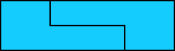All L-shaped polyominoes with a width of 2 can tile a rectangle.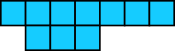We do not know if this polyomino tiles a rectangle.

The following set of polyominoes can tile any region other than the monomino. These are the only polyominoes that cannot be split into two smaller polyominoes where one is not the monomino.

The book covers many more topics, and delves deeper into the ideas sampled above. If you read it and have any feedback, I would love to hear it.